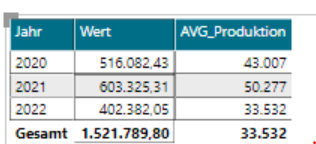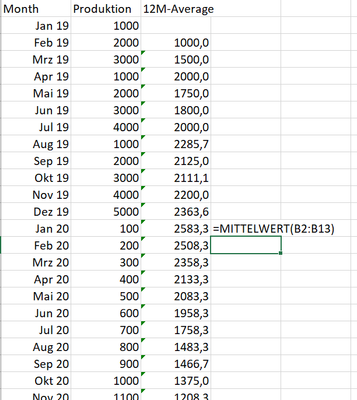cancel
Showing results for
Did you mean:Helper III

## Moving average / moving variance

Hi,
I want to calculate a moving average of my measure over the last 12 months.

I use the following DAX formula so far, which works, as long as my time granularity level is the month.

``````AVG_Produktion =
CALCULATE (
[PRODUKTION];
DATESINPERIOD (
Datumstabelle[Date];
LASTDATE ( Datumstabelle[Date] );
-12;
MONTH
)
) / 12
* DIVIDE ( [PRODUKTION]; [PRODUKTION] )``````

The results look like this:

The value for Jan 2022 is correct (average of the months Feb 2021 - Jan 2022), however the value for the whole year 2022 is obviously not correct - I'm actually not completely sure, where this value comes from.
What I want to see here is the sum of all the MA values for 2022, so that I can compare it to the actual yearly production.
Similarly, if I go down on the date level, I want to know, what the average daily production was in the last 12 months.I assume, there should be some kind of an elegant solution using the average or averagex function.

The other question is how to compute a moving variance for the same time periods, ideally without having to calculate the variance formula by hand, bur rather using the VARX.P function.

1 ACCEPTED SOLUTIONAnonymous
Not applicable

You are mixing 2 different measures. One is the 12-month average and the other is the sum of the 12-month averages. If you want to have these 2 morphed into one... then you'll need to write a SWITCH which will select the measure based on what time period is in scope.

Actually... on reflection, you can do something simpler: you can write a measure that will sum up the averages over the currently visible pieces of time. If you never go below the month level, then it's even easier. Hide the individual dates so that they can't be selected, leave only pieces of time from the month up and write:

sumx(
VALUES ( Calendar[YearMonth] ),

[12M Average]

)

This will give you what you want.

Best
D

6 REPLIES 6Super User

This looks like a measure totals problem. Very common. See my post about it here: https://community.powerbi.com/t5/DAX-Commands-and-Tips/Dealing-with-Measure-Totals/td-p/63376

Also, this Quick Measure, Measure Totals, The Final Word should get you what you need:
https://community.powerbi.com/t5/Quick-Measures-Gallery/Measure-Totals-The-Final-Word/m-p/547907

Become an expert!: Enterprise DNA
External Tools: MSHGQM
Latest book!:
Mastering Power BI 2nd EditionDAX is easy, CALCULATE makes DAX hard...Helper III

Hi Greg,
Thanks for your answer, but I guess this is not the problem in this case, though it may seem so.

If I just choose the year as my dimension, the value still remains the same, although there is no 'Total' lines involved.Anonymous
Not applicable

402.382 / 12 = 33.532.

The calculation is correct.

The Gesamt is correct as well according to the formula and to what one would expect. That's because it's not just an average but a 12-month monthly moving average.

Best
DHelper III

Hi,
maybe the term "moving average" is misleading or even wrong here. I'll try to illustrate what I want to have with a simple Excel example:

Imagine I have raw data per month. The 12M-moving average for January 2020 (=C14) is AVERAGE(B2:B13).  [or maybe Average(B3:B14, if you take the new value into the timeframe, there are varying definitions I guess]
With this formula, I can calculate the moving average for every month after the 12th.Now as long as I display this measure on the month level, everything is fine, I can simply display the calculated values without any further aggregation.
But if I choose to aggregate on the year level (or quarter, whatever), then I have to take the SUM of all the values in column C, not their average!
If I create a Pivot Table in Excel, this is exactly what happens. But I don't know how to achieve the same behaviour in PowerBI.Anonymous
Not applicable

You are mixing 2 different measures. One is the 12-month average and the other is the sum of the 12-month averages. If you want to have these 2 morphed into one... then you'll need to write a SWITCH which will select the measure based on what time period is in scope.

Actually... on reflection, you can do something simpler: you can write a measure that will sum up the averages over the currently visible pieces of time. If you never go below the month level, then it's even easier. Hide the individual dates so that they can't be selected, leave only pieces of time from the month up and write:

sumx(
VALUES ( Calendar[YearMonth] ),

[12M Average]

)

This will give you what you want.

Best
DHelper III

That looks good, thank you!

Although it didn't solve my intiial question on how to calculate the moving variance, accordingly, without having to write the variance formula explicitly. I hoped to find a way to calculate the desired moving average by using the function Averagex and adapt the filter settings to a different measure with the VARX.P function.